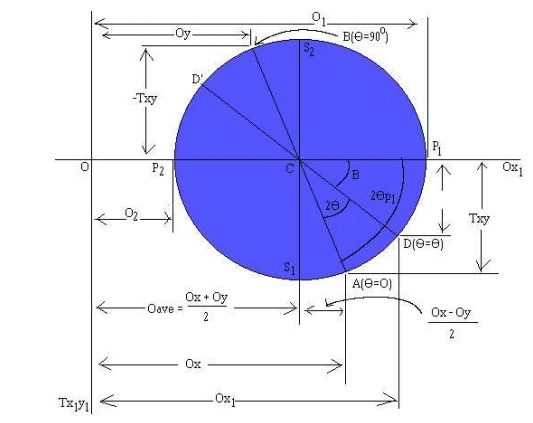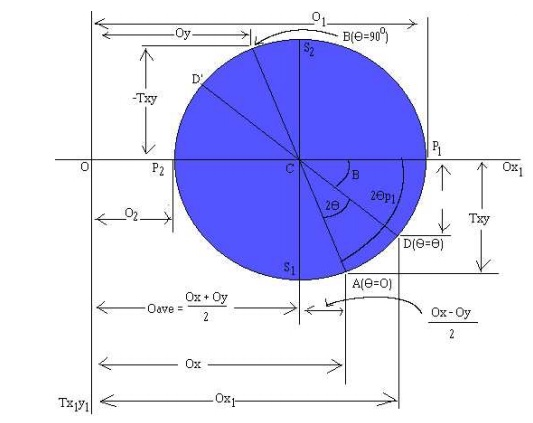Home | | Mechanics of Solids | Mohr's circle for biaxial stresses

# Mohr's circle for biaxial stressesTo find the maximum and minimum normal stresses throughout the entire range of angles, one can easily take the first derivative of with respect to theta, set it to zero, and solve for the angle.

Mohr's circle for biaxial stresses

To find the maximum and minimum normal stresses throughout the entire range of angles, one can easily take the first derivative of (3) with respect to theta, set it to zero, and solve for the angle. This will give what is called

the principal plane on which the principal stresses act. If this all sounds overly complicated... you're right! Why not just use the tried and true terminology "maximizing and minimizing the function" instead of inventing these two new terms with unrelated and unclear meaning? Well.... that's civil engineers for you.Where thetap defines the orientation of the principal planes, and its two values, differing by 180o, are called the principal angles.Now is where we begin to get into the unnecessary jargon. All the excess baggage some engineer created to make it so that utilizing these relationships would not require higher math. This (and many other examples of engineer idioticy) most likely stems from the fact that most engineers slept through their higher level math classes, and suffer from acute mathematical insecurities (and probably rightly so.) It's these abstract constructions which attempt to simplify the work, yet ultimately make it more difficult for those of us more mathematically inclined, that really piss me off. If you represent equation (5) geometrically with a 90o triangle, (left), we can obtain general formulas for the principal stresses. First, we note that the  hypotenuse of the triangle is,

R = SQR{ [(Ox - Oy)/2]2 + Txy2}  ----- (6)

The quantity R is defined as a positive number, and, like the other two sides of the triangle, has the completely meaningless units of "stress". From the triangle we obtain two additional relations:

cos(2thetap) = (Ox - Oy)/(2R)  sin(2thetap) = Txy/R      (7, 8)

Which is all very well and good, because it actually leads to the USEFUL equation for the general formula for the principal stresses:

O1,2 = (Ox + Oy)/2  +/- R - ------ (9)

But such usefulness is short lived as we approach MOHR'S CIRCLE..... Actually, Mohr's circle isn't all that bad in many cases. It supplies its practitioners a clever and easy way to compute otherwise hairy moments of inertia, allows strain analyses to be handled quickly. However, in this case, its application seems to me a bit of a stretch, and what you wind up with is this hopelessly complicated graphical representation that seems so much more difficult than the original equations (3) and (4) that it's hardly worth the effort to learn at all. HOWEVER....

because certain bastich elements in the civil engineering department here at the U of A are requiring their students (many of whom, myself included, will NEVER use these relationships again after the class has ended) to use this technique in spite of the fact that we know of a perfectly valid and correct alternative.

The equations of Mohr's circle can be derived from the transformation equations (3) and (4). By simply rearranging the first equation, we find that the two expressions comprise the equation of a circle in parametric form.

Ox1 - (Ox + Oy)/2 = [(Ox - Oy)/2]cos(2theta) + Txy sin(2theta)                (10)

Tx1y1 = - {(Ox - Oy)/2}sin(2theta) + Txy cos(2theta)           (11)

To eliminate the 2theta parameter, we square each relationship and add the two equations together. This ultimately leads to (after simplification),

(Ox1 - {Ox + Oy}/2)2 + Tx1y12 = {(Ox - Oy)/2}2 + Txy2          (12)

However, by resubstitution of equation (6) and by recognizing that the average stress value between the X and Y axis, Oave, is,

Oave = (Ox + Oy)/2                         (12.a)

equation (12) can be simplified into the semi friendly equation of a circle in standard algebraic form,

(Ox1 - Oave)2 + Tx1y12 = R2  (13)

However, don't let this nice looking equation for a circle fool you. Hidden in this simple equation are some of the most hairy, complicated, and down-right nasty relationships I think I have ever encountered. This makes my studies in the Frobenious theorem for solving differential equations with non-constant singular coefficients seem tame.With Ox, Oy, and Txy known, the procedure for constructing Mohr's circle is as follows:

1.     Draw a set of coordinate axis with Ox1 and Tx1y1 (with T positive downwards. From now on, for simplicity, O and T will represent their respective axis.)

2.     The center of the circle, by equation (13) is located at T=0 and O=Oave. Oave is nothing more than (12.a), so the center of the circle is located at:

C = (Ox + Oy)/2

3.     Locate point A, representing the stress conditions on the X face of the normal oriented element (Figure 1, extreme top left, non-rotated section). Plot coordinates O = Ox, T = Txy. Here, it is important to note that at point A, the inclination angle, theta, is zero.

4.     Locate point B, representing the stress conditions on the Y face of the normal oriented element (Figure 1, again, extreme top left, non-rotated section). Again, plot coordinates O = Oy, T = Txy. Note that this point, B, will be diametrically opposite from point A. Also note, that the angle of inclination at B, theta, will be 90o, as it could also be achieved on the X face by rotating it by 90o.

5.     Draw a line from point A to point B through the center C. This line is a diameter of the circle.

6.     Using point C as the center, draw Mohr's circle through points A and B. The circle will have a radius of R, which is the same R as in equation (6).

Now that you have Mohr's circle drawn, you can use it to analyze the problem. (Remember, that this method is every bit as valid as simply using equations (3) and (4) above, except it requires less mathematical skill, and many more memorized relationships.)

O1,2, representing the maximum and minimum normal stresses and their respective angles away from point A (where theta = 0o) can be found by simply looking at the O values when T = 0. In the drawing above,

O1 represents the maximum, and O2 the minimum. Furthermore, Tmax/min, representing the maximum and minimum shear stresses and their respected angles can be found by locating the T values when O = Oave. At this point, T is simply equal to the radius, R, or equation (6).

In addition to these helpful points, all other possible points for the shear and normal stresses can be found on this circle. In order to find another value of Ox, Oy for a given rotation, one must simply start at the A and B points (A representing the Ox value and B, the Oy value), and rotate in a positive theta direction (by the orientation shown above, this is in a counterclockwise direction, in keeping with the right hand rule) for 2theta (from equations (3) and (4) above). The resulting points, D and D', will yield the Ox, Txy, and Oy, Txy (respectively) for that rotation.

As I have likely mentioned before (likely because, I can't really recall) to me this seems all very abstract and difficult to use. However, the aforementioned bastiches will be requiring this on my upcoming test, so I felt a need to more fully understand it. Granted, I still don't understand it as fully as I would hope, but it ought to be enough to get me through this one, insignificant little test.

P.S.: I apologize for my editorializing and opinionated presentation of this topic. I rarely do this when I analyze problems I don't understand (even when I do not like the method, such as the Lewis Dot structure). This time, however, I have some very strong feelings about my predicament. Also, in all fairness, if you were given the problem where O1 = O2 and Tmax = 0, i.e. the Mohr's circle was simply a little dot with R = 0, using the Mohr's circle method would arrive you at any and all answers much quicker than using equations (3) and (4). However, I don't think this extreme simplification of one special case warrants the abstraction being a required bit of knowledge for civil engineers.

Study Material, Lecturing Notes, Assignment, Reference, Wiki description explanation, brief detail
Civil : Mechanics Of Solids : Thin Cylinders, Spheres And Thick Cylinders : Mohr's circle for biaxial stresses |